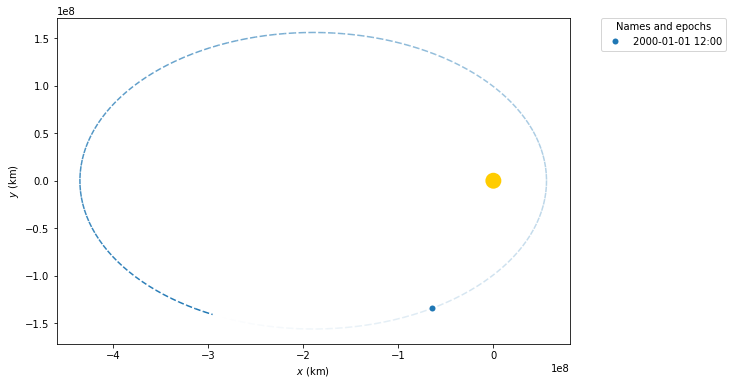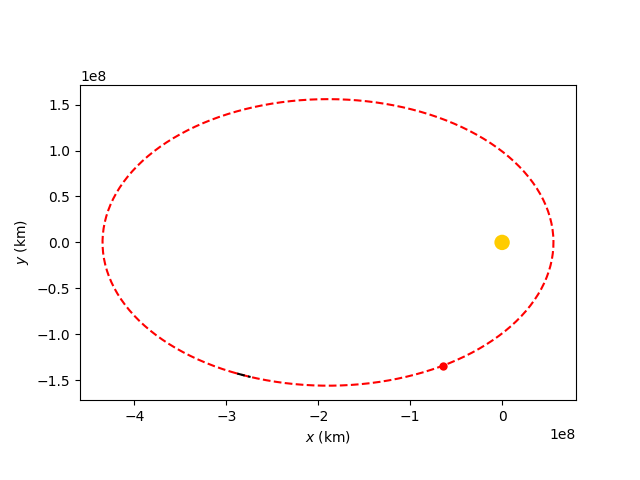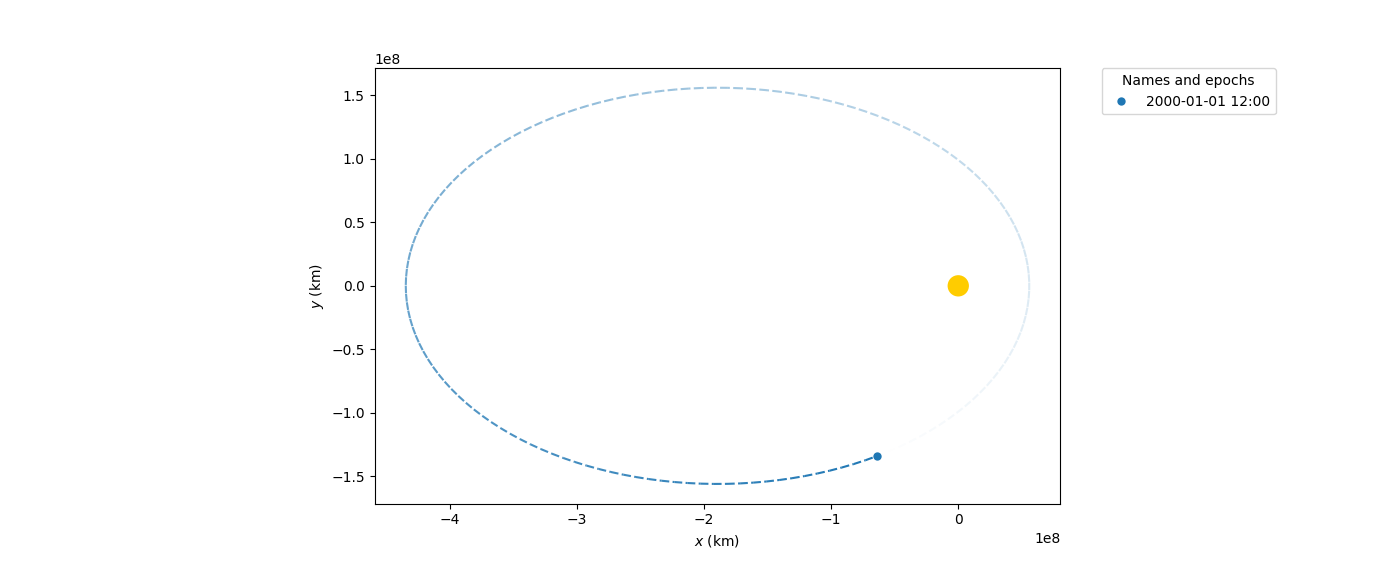{{ message }}
/ poliastro Public

# Propagation is inconsistent sometimes#901

Closed
opened this issue Apr 11, 2020 · 8 comments · Fixed by #926
Closed

# Propagation is inconsistent sometimes#901

opened this issue Apr 11, 2020 · 8 comments · Fixed by #926
Labels

###astrojuanlu commented Apr 11, 2020

 For example: ``````op = StaticOrbitPlotter() op.plot( Orbit.from_vectors( Sun, [0.36137779, 0.84843643, 0.36772403] * u.au, [-0.01849308, -0.00788258, -0.00339819] * u.au / u.day, ), trail=True, ) ``````The text was updated successfully, but these errors were encountered:

###astrojuanlu commented Apr 11, 2020

 Interestingly, this does not happen only with trail plotting. Take this snippet: ```from astropy import units as u from astropy.time import Time import matplotlib.pyplot as plt from poliastro.bodies import Sun, Earth, Mars from poliastro.twobody import Orbit from poliastro.twobody.propagation import propagate from poliastro.maneuver import Maneuver from poliastro.plotting.static import StaticOrbitPlotter from poliastro.util import time_range ss = Orbit.from_vectors( Sun, [0.36137779, 0.84843643, 0.36772403] * u.au, [-0.01849308, -0.00788258, -0.00339819] * (u.au / u.day) ) coordinates = propagate( ss, time_range(ss.epoch, periods=200, end=ss.epoch + 10 * u.day), ) fig, ax = plt.subplots() op = StaticOrbitPlotter(ax=ax) op.set_attractor(Sun) op.set_frame(*ss.pqw()) op.plot(ss, color="r"); op.plot_trajectory(coordinates, color="k") ax.set_xlim(-459279554.4666631, 80427317.98609273) ax.set_ylim(-171462462.17020893, 171486927.37946865)```Explanation: In red, the fully sampled orbit, and a big red dot where the actual position is at the given epoch I am taking that `Orbit`, called `ss`, and calling the `propagate` function on it on a time range that starts at `ss.epoch` and starts a bit later to see if the small trace matches the position of the big red dot ...and it's not happening. There's something weird going on here.changed the title Trail plotting is inconsistent sometimes Trajectory plotting is inconsistent sometimes Apr 11, 2020

###astrojuanlu commented Apr 11, 2020

 This can be observed in poliastro 0.13.1.

Merged

###priyanshurohilla commented Apr 12, 2020

 I think this is happening because you have not propagated the `epoch` . You have extracted the coordinates but did not propagated the epoch and i think maybe that's why the red marker has not changed the position.

###Meuge commented Apr 12, 2020

 Hi @priyanshurohilla ! I’ve recently talked with @astrojuanlu about the issue, and it seems the problem is with the transpose in the plot coordinates. Thanks for the suggestion :). I’ll also consider it while solving the issue.

###Meuge commented Apr 20, 2020

 It seems the problem comes from the propagate method as there is a discrepancy in the position values. As you may notice from the example below: ``````from poliastro.bodies import Earth from poliastro.twobody import Orbit from poliastro.twobody.propagation import propagate position = [-6045, -3490, 2500] * u.km velocity = [-3.457, 6.618, 2.533] * u.km / u.s ss = Orbit.from_vectors(Earth, position, velocity) coordinates = propagate(ss,ss.epoch.reshape(1)) print(coordinates) print(ss.rv()) #The one define in position `````` Output `````` [(-7010.52954739, 1276.67143012, 3581.24784291)] km [-6045. -3490. 2500.] km ``````

###astrojuanlu commented Apr 20, 2020

 Thanks @Meuge! This has nothing to do with plotting then, so changing the title accordingly.changed the title Trajectory plotting is inconsistent sometimes Propagation is inconsistent sometimes Apr 20, 2020

###astrojuanlu commented Apr 21, 2020

 Another way to reproduce this issue: ```from astropy import units as u from astropy.time import Time import matplotlib.pyplot as plt from poliastro.bodies import Sun from poliastro.twobody import Orbit from poliastro.plotting.static import StaticOrbitPlotter orb = Orbit.from_vectors( Sun, [0.36137779, 0.84843643, 0.36772403] * u.au, [-0.01849308, -0.00788258, -0.00339819] * u.au / u.day, ) op = StaticOrbitPlotter() op.plot(orb) op.plot_trajectory(orb.sample(min_anomaly=orb.nu, max_anomaly=orb.nu + 5 * u.deg), color="k") plt.show()``` Probably because: ``````In : orb.r.to(u.km) Out: In : orb.sample(1, min_anomaly=orb.nu, max_anomaly=orb.nu + 1e-3 * u.deg).get_xyz(xyz_axis=1).to(u.km) Out: ``````

added a commit to astrojuanlu/poliastro that referenced this issue Apr 22, 2020
``` Fix sampling logic for closed orbits ```
``` e294e47 ```
`Fix poliastro#901.`
added a commit to astrojuanlu/poliastro that referenced this issue Apr 22, 2020
``` Fix sampling logic for closed orbits ```
``` 0e19184 ```
`Fix poliastro#901.`
Refactor propagators automation moved this from To do to Done Apr 22, 2020

###astrojuanlu commented Apr 22, 2020

 Original figure after merging #926:✔️